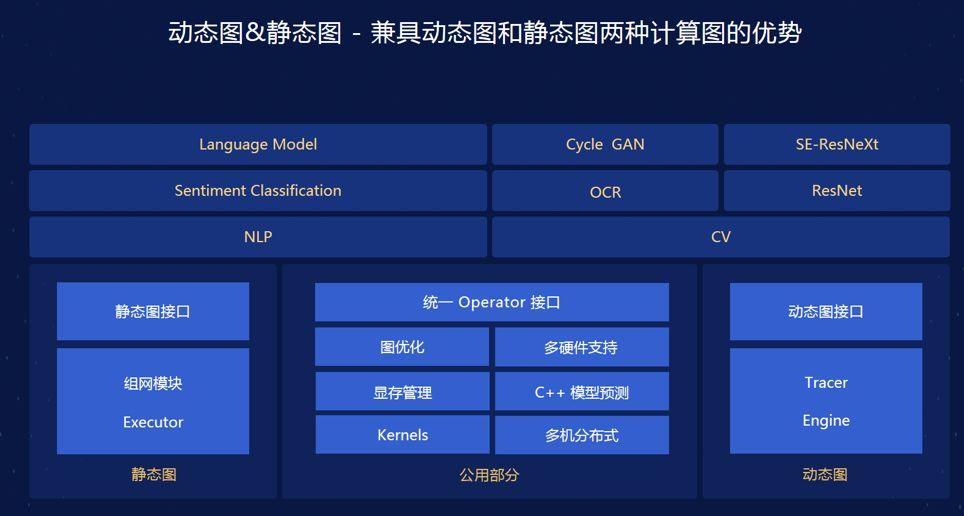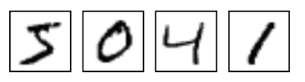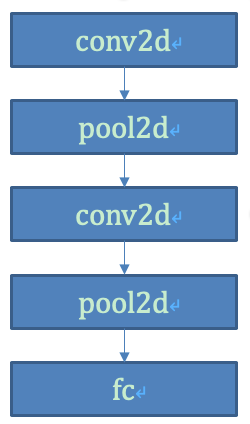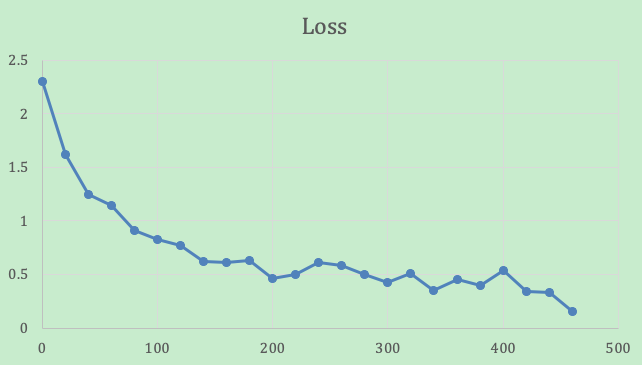# 都9102年了还不懂动态图吗？一文带你了解飞桨动态图

2020/03/20 01:27

下载安装命令

## CPU版本安装命令

## GPU版本安装命令
pip install -f https://paddlepaddle.org.cn/pip/oschina/gpu paddlepaddle-gpu# 2. 飞桨动态图的三大特色

• 灵活便捷的代码书写方式：能够使用Python的控制流（for，if…else..等）进行编程。

• 便捷的调试功能：直接使用Python的打印方法即时打印所需要的结果，从而检查正在运行的模型结果便于调试。

• 和静态执行图通用的模型代码：对于没有使用Python控制流的网络，动态图的代码可以直接在静态图模式下执行，提升执行的效率。

# 3. 飞桨动态图与静态图的直观对比

（1）  如果inp1各元素之和小于inp2各元素之和，那么执行inp1与 inp2各元素对应相加。

（2）  如果inp1各元素之和大于等于inp2各元素之和，那么执行inp1与 inp2各元素对应相减。

import paddle.fluid asfluid
import numpy as np
inp1 = np.random.rand(4, 3, 3)
inp2 = np.random.rand(4, 3, 3)
# dynamic graph
with fluid.dygraph.guard():
if np.sum(inp1) &lt;np.sum(inp2):
else:
x =fluid.layers.elementwise_sub(inp1, inp2)
dygraph_result = x.numpy()

import paddle.fluid asfluid
import numpy as np
inp1 = np.random.rand(4, 3, 3)
inp2 = np.random.rand(4, 3, 3)
# static graph
with new_program_scope():
inp_data1 =fluid.layers.data(name='inp1', shape=[3, 3], dtype=np.float32)
inp_data2 =fluid.layers.data(name='inp2', shape=[3, 3], dtype=np.float32)

a =fluid.layers.expand(fluid.layers.reshape(fluid.layers.reduce_sum(inp_data1),[1, 1]), [4, 1])
b =fluid.layers.expand(fluid.layers.reshape(fluid.layers.reduce_sum(inp_data2),[1, 1]), [4, 1])
cond =fluid.layers.less_than(x=a, y=b)

ie =fluid.layers.IfElse(cond)
with ie.true_block():
d1 =ie.input(inp_data1)
d2 =ie.input(inp_data2)
ie.output(d3)

with ie.false_block():
d1 =ie.input(inp_data1)
d2 =ie.input(inp_data2)
d3 =fluid.layers.elementwise_sub(d1, d2)
ie.output(d3)
out = ie()

exe =fluid.Executor(fluid.CPUPlace() if not core.is_compiled_with_cuda() elsefluid.CUDAPlace(0))
static_result =exe.run(fluid.default_main_program(),feed={'inp1': inp1,'inp2':inp2},fetch_list=out)

# 4. 飞桨动态图的基本用法

（1）  动态图与静态图的最大区别是采用了命令式的编程方式，任务不用在区分组网阶段和执行阶段。代码运行完成之后，可以立马获取结果。由于采用与我们书写大部分Python和c++的方式是一致的命令式编程方式，程序的编写和调试会非常的容易。

（2）  同时动态图能够使用Python的控制流，例如for,if else, switch等，对于rnn等任务的支持更方便。

（3）  动态图能够与numpy更好的交互。

pip install -q --upgrade paddlepaddle==1.5.1
with fluid.dygraph.guard():

Dygraph非常适合和Numpy一起使用，使用fluid.dygraph.to_variable(x)将会将Numpy的ndarray转换为fluid.Variable，而使用fluid.Variable.numpy()将可以把任意时刻获取到的计算结果转换为Numpy ndarray，举例如下：

import paddle.fluid asfluid
import numpy as np
x = np.ones([10, 2, 2], np.float32)

with fluid.dygraph.guard():
inputs = []
seq_len = x.shape
for i in range(seq_len):
inputs.append(fluid.dygraph.to_variable(x[i]))
ret =fluid.layers.sums(inputs)
print(ret.numpy())  

   [[10. 10.]
[10. 10.]]           

    loss =fluid.layers.reduce_sum(ret)
loss.backward()
print(loss.gradient())

   [1.]

5. 飞桨动态图的项目实战

MNIST示例代码地址：## 5.1   数据准备

train_reader = paddle.batch(paddle.dataset.mnist.train(),batch_size=BATCH_SIZE, drop_last=True)

## 5.2  Layer定义

Conv2D是飞桨提供的卷积运算的Layer，Pool2D是池化操作的Layer。

1）定义SimpleImgConvPool 子Layer：SimpleImgConvPool把网络中循环使用的部分进行整合，其中包含包含了两个子Layer，Conv2D和Pool2D，forward函数定义了前向运行时的结构。

class SimpleImgConvPool(fluid.dygraph.Layer)
def __init__(self,name_scope, num_filters, filter_size, pool_size, pool_stride, pool_padding=0, pool_type='max',global_pooling=False, conv_stride=1, conv_padding=0, conv_dilation=1, conv_groups=1,act=None, use_cudnn=False, param_attr=None, bias_attr=None):
super(SimpleImgConvPool,self).__init__(name_scope)
def forward(self,inputs):
x =self._conv2d(inputs)
x = self._pool2d(x)
return x 

2）构建MNIST Layer，MNIST Layes包含了两个SimpleImgConvPool子Layer,以及一个FC（全连接层），forward函数定义了如图2所示得网络结构

class MNIST(fluid.dygraph.Layer):
def __init__(self,name_scope):
super(MNIST,self).__init__(name_scope)
self._simple_img_conv_pool_1 = SimpleImgConvPool(self.full_name(), 20,5, 2, 2, act="relu")
self._simple_img_conv_pool_2 = SimpleImgConvPool(self.full_name(), 50,5, 2, 2, act="relu")
pool_2_shape = 50 *4 * 4
SIZE = 10
scale = (2.0 / (pool_2_shape**2 *SIZE))**0.5
self._fc =fluid.dygraph.FC(self.full_name(),10, param_attr=fluid.param_attr.ParamAttr(initializer=fluid.initializer.NormalInitializer(loc=0.0, scale=scale)),act="softmax")
def forward(self, inputs,label=None):
x =self._simple_img_conv_pool_1(inputs)
x =self._simple_img_conv_pool_2(x)
x = self._fc(x)
if label is notNone:
acc =fluid.layers.accuracy(input=x, label=label)
return x, acc
else:
return x

## 5.3  优化器定义

adam =fluid.optimizer.AdamOptimizer(learning_rate=0.001)

## 5.4   训练

with fluid.dygraph.guard():
epoch_num = 5
BATCH_SIZE = 64

mnist =MNIST("mnist")

np.set_printoptions(precision=3,suppress=True)
for epoch inrange(epoch_num):
dy_x_data = np.array(
[x.reshape(1, 28, 28)
for x indata]).astype('float32')
y_data =np.array(
[x for xin data]).astype('int64').reshape(BATCH_SIZE, 1)
img =fluid.dygraph.to_variable(dy_x_data)
label =fluid.dygraph.to_variable(y_data)
cost =mnist(img)
loss =fluid.layers.cross_entropy(cost, label)
avg_loss =fluid.layers.mean(loss)
dy_out =avg_loss.numpy()
avg_loss.backward()
dy_param_value ={}
for param inmnist.parameters():
dy_param_value[param.name] = param.numpy()
if batch_id % 20== 0:
print("Loss at step {}: {}".format(batch_id,avg_loss.numpy()))

## 5.5  预测

def test_mnist(reader, model, batch_size):
acc_set = []
avg_loss_set = []
dy_x_data = np.array([x.reshape(1, 28, 28) for x indata]).astype('float32')
y_data = np.array([x for x indata]).astype('int64').reshape(batch_size, 1)
img = to_variable(dy_x_data)
label = to_variable(y_data)
prediction, acc = model(img, label)
loss = fluid.layers.cross_entropy(input=prediction, label=label)
avg_loss = fluid.layers.mean(loss)
acc_set.append(float(acc.numpy()))
avg_loss_set.append(float(avg_loss.numpy()))
# get test acc and loss
acc_val_mean = np.array(acc_set).mean()
avg_loss_val_mean = np.array(avg_loss_set).mean()
return avg_loss_val_mean, acc_val_mean## 5.6   调试

• 动态图代码地址：下载安装命令

## CPU版本安装命令

## GPU版本安装命令
pip install -f https://paddlepaddle.org.cn/pip/oschina/gpu paddlepaddle-gpu

0
0 收藏

### 作者的其它热门文章0 评论
0 收藏
0